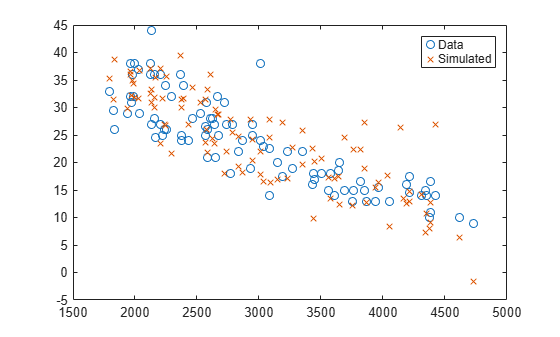random

Simulate responses with random noise for linear regression model

Description

example

ysim = random(mdl,Xnew) simulates responses to the predictor data in Xnew using the linear model mdl, adding random noise.

Examples

collapse all

Create a quadratic model of car mileage as a function of weight from the carsmall data set.

X = Weight;
y = MPG;

Create simulated responses to the data with random noise.

ysim = random(mdl,X);

Plot the original responses and the simulated responses to see how they differ.

plot(X,y,'o',X,ysim,'x')
legend('Data','Simulated')Input Arguments

collapse all

Linear regression model object, specified as a LinearModel object created by using fitlm or stepwiselm, or a CompactLinearModel object created by using compact.

New predictor input values, specified as a table, dataset array, or matrix. Each row of Xnew corresponds to one observation, and each column corresponds to one variable.

• If Xnew is a table or dataset array, it must contain predictors that have the same predictor names as in the PredictorNames property of mdl.

• If Xnew is a matrix, it must have the same number of variables (columns) in the same order as the predictor input used to create mdl. Note that Xnew must also contain any predictor variables that are not used as predictors in the fitted model. Also, all variables used in creating mdl must be numeric. To treat numerical predictors as categorical, identify the predictors using the 'CategoricalVars' name-value pair argument when you create mdl.

Data Types: single | double | table

Output Arguments

collapse all

Simulated response value, returned as a numeric vector. The simulated value is the predicted response values at Xnew perturbed by random noise. The noise is independent and normally distributed, with mean equal to zero and variance equal to the estimated error variance of the model.

Alternative Functionality

For predictions without random noise, use predict or feval. These two functions give the same predictions.

• predict accepts a single input argument containing all predictor variables, and gives confidence intervals on its predictions.

• feval accepts multiple input arguments with one input for each predictor variable.

Extended Capabilities

Introduced in R2012a